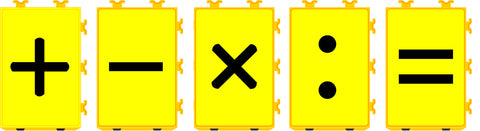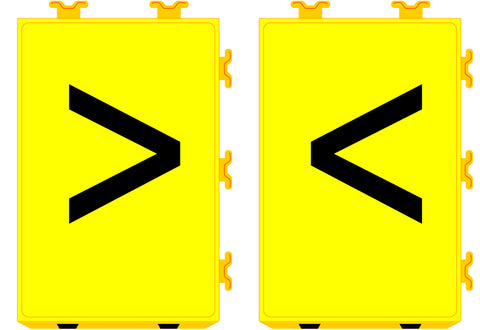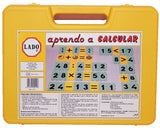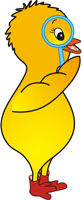# We continue, Operations on the run

As we agreed a few days ago, today in our article we provide you with exercises already proposed to Play: "Operations on the run" with I Learn to Calculate (besides we offer you a discount for everyone who wants to have it)

We have classified the exercises by the difficulty of the operations:

simple :1º Simple operation (addition and subtraction) and non-repeated results

Row of Operations: 1+2=, 4+4=, 5+1=,3+2=, 5-3=, 8+2=, 7-4=, 6+3=, 3+8=, 1 +6= Results: 3,8,6,5,2,10,3,9,11,7
Objective: For each child with the result sheet to find their partner. I encourage speed, whoever arrives before wins 1 point.

2nd Simple operation (multiplication) and non-repeated results

Row of Operations: 1x2=, 4x2=, 5x3=,3x2=, 3x1=, 2x2=, 3x3=, 7x1=, 3x1=, 5x62 Results: 2,8,15,6,3,4,9,7, 3.10
Objective: For each child with the result sheet to find their partner. I encourage speed, whoever arrives before wins 1 point.
3rd Simple operation (multiplication and division) and non-repeated results
Row of Operations: 4x1=, 8x2=, 5:1=,6:2=, 3x4=, 2:2=, 5x2=, 2x3=, 3x5=, 6:3= Results:4,16,5,3 ,12,1,10,6,15,2
Objective: For each child with the result sheet to find their partner. I encourage speed, whoever arrives before wins 1 point.

More complicated by using 2-digit numbers :4th 2-digit numbers (multiplication and division) and non-repeated results
Operation Row: 10x5=, 30:3=, 15:3=,16:2=, 11x4=, 22:2=, 16:4=, 18:9=, 3x7=, 36:3= Results:50 ,10,5,8,44,11,4,2,21,12
Objective: For each child with the result sheet to find their partner. I encourage speed, whoever arrives before wins 1 point.
More competitive:
5º Now there is one less operation, and repeated results , who will be left without a partner ?
Row of Operations (attention there are only 9): 50:5=, 7+3=, 5x3=, 8x4=, 22-1=, 16:4=, 27:9=, 3x7=, 36:3=
Results (Be careful we put 10 results) :10,10,15,32,21,4,3,21,12,10
Objective: For each child with the result sheet to find their partner. Incentive to get a partner. Whoever arrives last will be left without a partner.

More complicated Concepts and Operations:6º We now use the fractions and their symbols.
Operation Row: 1/2=, 1/4= , 3/4= , 1/2x1/2= , 4/4
Results: Half Symbol, Quarter Symbol, 3 Quarter Symbol, Half Symbol, Unit Symbol
Objective: For each child with the result sheet to find their partner. I encourage speed, whoever arrives before wins 1 point.

7º We use the symbols of greater and lesser and it is necessary to see which of the answers meet the criteria of each question.Row of Operations: Is <9 and >2 , Is >10 and <12, Is <3 and >1, Is >8 and <11

Results: 3-4-5-6-7-8, 11, 2, 9-10

Objective: For each child with a result sheet to look for the criteria in which their result fits.

With this we have given you several examples so that you can apply them directly in your classrooms, motivating children to mental calculation and working on mathematical concepts not only on paper.

Thanks for reading, see you in the next article.

PS: If you still don't have it and you are interested in having your Aprendo a Calcular, get it now with a 5% discount for following us. Promotion Code: OPERACIONES_CARRERA

Valid until Friday, April 7, 2017.